Why does 1 3/4 + 2 9/10 equal 4.65?
How do you solve this?

Correct result:

x =  4.65

#### Solution:

$a=1 + \dfrac{ 3 }{ 4 }=\dfrac{ 7 }{ 4 }=1.75 \ \\ b=2 + \dfrac{ 9 }{ 10 }=\dfrac{ 29 }{ 10 }=2.9 \ \\ \ \\ x=a+b=1.75+2.9=\dfrac{ 93 }{ 20 }=4.65$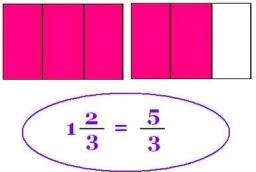We would be very happy if you find an error in the example, spelling mistakes, or inaccuracies, and please send it to us. We thank you!Tips to related online calculators
Need help calculate sum, simplify or multiply fractions? Try our fraction calculator.

## Next similar math problems:

• FractionFraction ? write as fraction a/b, a, b is integers numerator/denominator.
• DiophantusWe know little about this Greek mathematician from Alexandria, except that he lived around 3rd century A.D. Thanks to an admirer of his, who described his life by means of an algebraic riddle, we know at least something about his life. Diophantus's youth
• Say we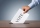Say we have 70 voting delegates and we need a 2/3 vote What would that number be? Also same as above but in this virtual world, it converts to percentages. What would the % number of positive votes be?
• Collection of stampsJano, Rado, and Fero have created a collection of stamps in a ratio of 5: 6: 9. Two of them had 429 stamps together. How many stamps did their shared collection have?
• Youth trackYouth track from Hronská Dúbrava to Banská Štiavnica which announced cancellation attracted considerable media attention and public opposition, has cost 6.3 euro per capita and revenue 13 cents per capita. Calculate size of subsidies to trip group of 28 s
• Camp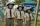In the camp are children. 1/2 went on a trip, 1/4 went to bathe and 38 children remained in the room. How many children are in camp?
• Drawing from a hatWhen drawing numbers from a hat from 1 to 35, we select random given numbers. What is the probability that the drawn numbers will be divisible by 8 and 2?
• My fatherMy father has a big farm. 6/8 of it were planted with mango trees, 1/2 of the remainder are guava trees and the rest 10 trees are santol trees. What is the number of all trees?
• Four pupilsFour pupils divided $1485 so that the second received 50% less than the first, the third 1/2 less than a fourth and fourth$ 154 less than the first. How much money had each of them?
• Ratio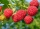Alena collected 7.8 kg of blueberries, 2.6 kg of blackberries, and 3.9 kg of cranberries. Express the ratio in the smallest natural numbers in this order.
• Closest natural numberFind the closest natural number to 4.456 to 44.56 and to 445.6.
• BoatsThree-quarters of boats are white, 1/7 are blue and 9 are red. How many boats do we have?
• Homework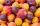In the crate are 18 plums, 27 apricot and 36 nuts. How many pieces of fruit left in the crate when Peter took 8 ninth: 1. nuts 2. apricots 3. fruit 4. drupe
• The number 72The number 72 increase by 25%. By how much % will you have to reduce the number you created to get the number 72 again?
• Job applicantsJob applicants: three-fourths of applicants had experience for a position. The number that did not have prior experience was 36. How many people applied for the job?
• LibraryNew books were purchased for the library. Five-eighths were professional books, one-fifth were encyclopedias, and 231 books were dictionaries. How many professional books were there?
• Quarantine cupcakes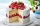Mr. Honse was baking quarantine cupcakes. Mrs. Carr made twice as many as Mr. Honse. Ms. Sanchez made 12 cupcakes more than Mr. Honse. If they put all their cupcakes together (which they can’t because. .. quarantine!) they would have 108 cupcakes. How may